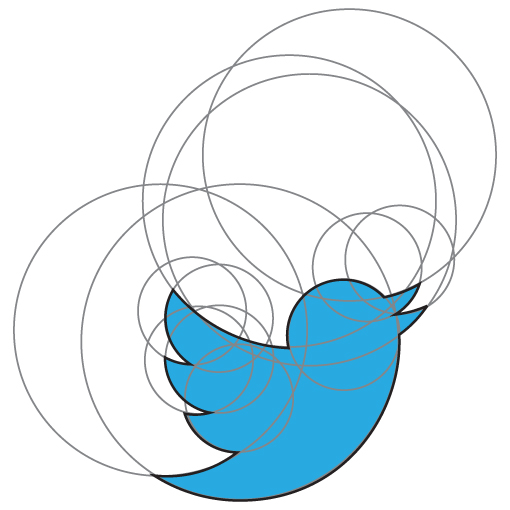# The Twitter Logo As SVG Circles

by | | Read ~1,221 times.

Five years ago, Twitter updated its logo and demonstrated that it could be made entirely of circles.Interestingly, the official Twitter logo doesn't use circles directly.

So, here is an SVG I've made which is only circles:

Or, without the edges, and no transparency:

And the raw code:

``````<svg height="100" viewBox="0 0 100 100" width="100" xmlns="http://www.w3.org/2000/svg">
<circle cx="50" cy="50"  fill="#fff"    r="100"/>
<circle cx="35" cy="33"  fill="#1da1f3" r="56"/>
<circle cx="07" cy="29"  fill="#fff"    r="51"/>
<circle cx="35" cy="53"  fill="#1da1f3" r="19"/>
<circle cx="23" cy="39"  fill="#fff"    r="20"/>
<circle cx="27" cy="40"  fill="#1da1f3" r="19"/>
<circle cx="18" cy="23"  fill="#fff"    r="19"/>
<circle cx="27" cy="24"  fill="#1da1f3" r="19"/>
<circle cx="53" cy="39"  fill="#1da1f3" r="19"/>
<circle cx="52" cy="-17" fill="#fff"    r="51"/>
<circle cx="84" cy="14"  fill="#1da1f3" r="17"/>
<circle cx="91" cy="05"  fill="#fff"    r="19"/>
<circle cx="80" cy="07"  fill="#1da1f3" r="19"/>
<circle cx="73" cy="-32" fill="#fff"    r="51"/>
<circle cx="72" cy="31"  fill="#1da1f3" r="19"/>
</svg>
``````

The eagle-eyed of you will notice that the lower beak is a different circle - and there's an extra one in the middle. Can you work out a better way to arrange them?

(I've recently become a little obsessed with SVGs.)